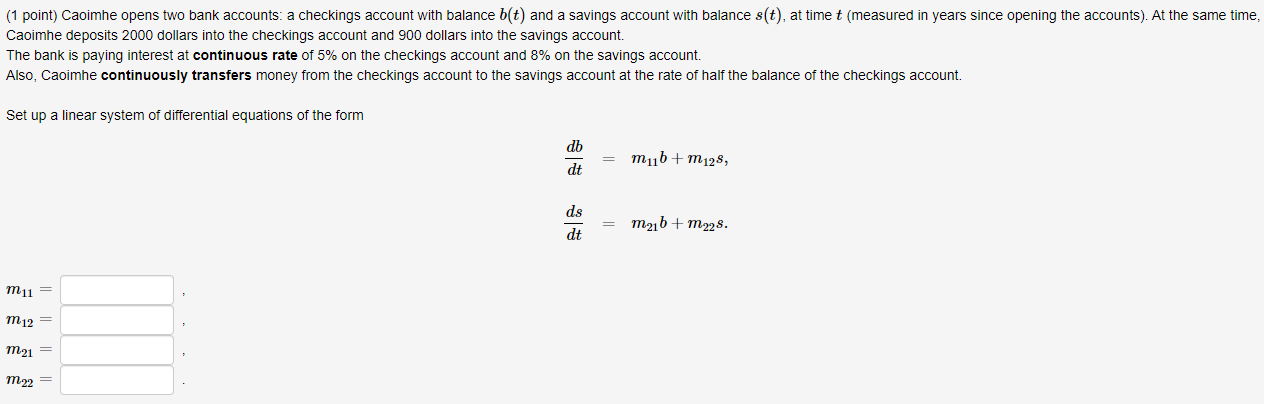# Question Hi, can anybody help on this question?Thanks in advance! (1 point) Caoimhe opens two bank accounts: a checkings account with balance b(t) and a savings account with balance s(t), at time t (measured in years since opening the accounts). At the same time, Caoimhe deposits 2000 dollars into the checkings account and 900 dollars into the savings account. The bank is paying interest at continuous rate of 5% on the checkings account and 8% on the savings account. Also, Caoimhe continuously transfers money from the checkings account to the savings account at the rate of half the balance of the checkings account. Set up a linear system of differential equations of the form db mub+m128, dt ds M21b + m228. dt m 11 = m 12 = m2 = m22 =Hi, can anybody help on this question?Transcribed Image Text: (1 point) Caoimhe opens two bank accounts: a checkings account with balance b(t) and a savings account with balance s(t), at time t (measured in years since opening the accounts). At the same time, Caoimhe deposits 2000 dollars into the checkings account and 900 dollars into the savings account. The bank is paying interest at continuous rate of 5% on the checkings account and 8% on the savings account. Also, Caoimhe continuously transfers money from the checkings account to the savings account at the rate of half the balance of the checkings account. Set up a linear system of differential equations of the form db mub+m128, dt ds M21b + m228. dt m 11 = m 12 = m2 = m22 =
More
Transcribed Image Text: (1 point) Caoimhe opens two bank accounts: a checkings account with balance b(t) and a savings account with balance s(t), at time t (measured in years since opening the accounts). At the same time, Caoimhe deposits 2000 dollars into the checkings account and 900 dollars into the savings account. The bank is paying interest at continuous rate of 5% on the checkings account and 8% on the savings account. Also, Caoimhe continuously transfers money from the checkings account to the savings account at the rate of half the balance of the checkings account. Set up a linear system of differential equations of the form db mub+m128, dt ds M21b + m228. dt m 11 = m 12 = m2 = m22 =